## Statement

Consider a quadratic equation of the form: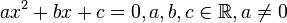$ax^2 + bx + c = 0, a,b,c \in \R, a \ne 0$

This is treated as an equation in$x$. The quadratic formula is a formula to determine the solutions of this equation. The short version of the formula is that the roots are:$\frac{-b \pm \sqrt{b^2 - 4ac}}{2a}$

if the expression makes sens; otherwise there are no roots.

To understand the formula, first define the discriminant of the quadratic function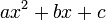$ax^2 + bx + c$ as the value$b^2 - 4ac$. Now, we make three cases:

Case for discriminant$b^2 - 4ac$ Conclusion for roots Conclusion for factorization of polynomial$ax^2 + bx + c$
positive, i.e.,$b^2 - 4ac > 0$ there are two real roots, given as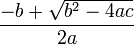$\frac{-b + \sqrt{b^2 - 4ac}}{2a}$ and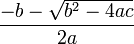$\frac{-b - \sqrt{b^2 - 4ac}}{2a}$ If we denote the roots by$\alpha, \beta$, then$ax^2 + bx + c = a(x- \alpha)(x - \beta)$
zero, i.e.,$b^2 - 4ac = 0$ there is a single real root with multiplicity two, and that root is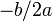$-b/2a$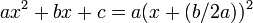$ax^2 + bx + c = a(x + (b/2a))^2$
negative, i.e.,$b^2 - 4ac < 0$ there are no real roots the polynomial does not factor, i.e., it is irreducible